## Example Questions

← Previous 1 3 4 5 6 7 8 9 48 49

### Example Question #1 : Algebra

What is the value of t if:  3x2 + tx - 21 = (3x - 3)(x + 7)?

-3
18
24
-18
21
Explanation:

Use the foil method:  (3x - 3) (x + 7) = 3x2 +21x - 3x - 21 = 3x2 +18x -21 so t = 18.

### Example Question #1 : Algebra

Expand the following equation: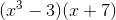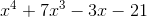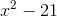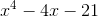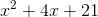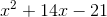Explanation:

use FOIL to factor the expression.

First: (x3)(x) = x4

Outside (x3)(7) = 7x3

Inside (–3)(x) = –3x  (Don't forget the negatives!)

Last (3)(7) = –21

### Example Question #1 : Algebra

Quantitative Comparison

Quantity A: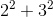Quantity B: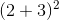The two quantities are equal.

Quantity B is greater.

Quantity A is greater.

The relationship cannot be determined from the information given.

Quantity B is greater.

Explanation:

Quantity A: 22 + 32 = 4 + 9 = 13

Quantity B: (2 + 3)2 = 52 = 25, so Quantity B is greater.

We can also think of this in more general terms.  x2 + y2 does not generally equal (x + y)2.

### Example Question #1 : How To Use Foil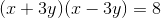Quantity A: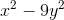Quantity B:Quantity B is greater.

The relationship cannot be determined from the information given.

The two quantities are equal.

Quantity A is greater.

Quantity B is greater.

Explanation:

The difference of squares formula says (x + a)(x - a) = x2 - a2.

Thus, Quantity A equals 8.

Therefore, Quantity B is greater.

### Example Question #1 : Gre Quantitative Reasoning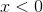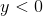Quantity A: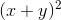Quantity B: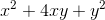Quantity B is greater.

The relationship cannot be determined.

The two quantities are equal.

Quantity A is greater.

Quantity B is greater.

Explanation:

To approach this problem, consider the two quantities

Quantity A:Quantity B:They are in different forms, so expand quantity A:

Quantity A: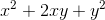Quantity B:Now, for the purpose of comparison, subtract shared terms from each quantity:

Quantity A*:Quantity B*: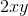Bothandare negative, non-zero values. Sinceis a product of two negative values, it must be positive. Quantity B must be greater than Quantity A.

### Example Question #1 : Gre Quantitative Reasoning

Expand the function: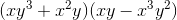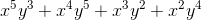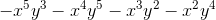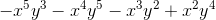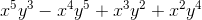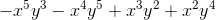Explanation:

Use the method of FOIL (First, Outside, Inside, Last) and add exponents for like bases: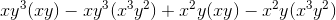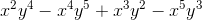### Example Question #1 : Algebra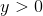Quantity A: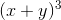Quantity B: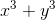Quantity A is greater.

The two quantities are equal.

The relationship cannot be determined.

Quantity B is greater.

The relationship cannot be determined.

Explanation:

Begin by expanding Quantity A: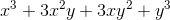Now in order to compare this to Quantity B:A good method would be to subtract shared terms from each Quantity; in this case, both quantities have an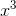and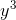term. Removing them gives:

Quantity A' :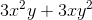Quantity B' :The question now is the sign of Quantity A'; if it's always positive, Quantity A is greater. If it's always negative, Quantity B is greater. If it is zero, the two are the same.

We only know thatIf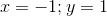, then Quantity A' would be zero.

If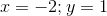, then Quantity A' would be positive.

Since values of x and y can be chosen to vary the relationship, th relationship cannot be determined.

### Example Question #1 : Algebra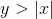Quantity A:Quantity B:The two quantities are equal.

Quantity A is greater.

Quantity B is greater.

The relationship cannot be determined.

Quantity B is greater.

Explanation:

Begin by expanding Quantity A:Now in order to compare this to Quantity B:A good method would be to subtract shared terms from each Quantity; in this case, both quantities have anandterm. Removing them gives:

Quantity A' :Quantity B' :The question now is the sign of Quantity A'; if it's always positive, Quantity A is greater. If it's always negative, Quantity B is greater. If it is zero, the two are the same.

We  know thatNow compare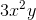and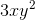Looking at absolute values so that we're only considering positive terms: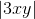From this it follows that by multiplyingacross the inequality :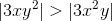From this we can determine that the magnitude of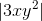is greater. However, since this is the product of one negative number and two positive numbers,is negative, and the sum ofandmust in turn be negative, and so Quantity A' must be negative!

From this we can say that Quantity B is greater.

### Example Question #1 : Algebra

Quantity A: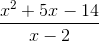Quantity B: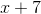Quantity A is greater.

The two quantities are equal.

The relationship cannot be determined.

Quantity B is greater.

The relationship cannot be determined.

Explanation:

This problem is deceptive. Looking at Quantity A, one may think to factor and reduce it as follows: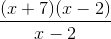Which is identical to Quantity B.

However, we cannot ignore that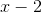in the original fraction! We are given no conditions as to the value of. If, then Quantity A would be undefined. Since we're not given the condition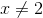, we cannot ignore this possibility.

The relationship cannot be established.

### Example Question #1 : Gre Quantitative Reasoning

Solve the following expression,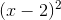.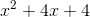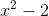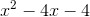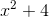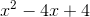Explanation:

You must FOIL the expression which means to multiply the first terms together followed by the outer terms, then the inner terms and lastly, the last terms.

The expression written out looks like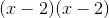.

You multiple both First terms to get.

Then the outer terms are multiplied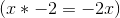.

Then you multiple the inner terms together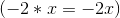.

Finally you multiply the last terms of each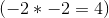.

This gives you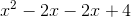or.

← Previous 1 3 4 5 6 7 8 9 48 49

### All GRE Math Resources